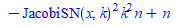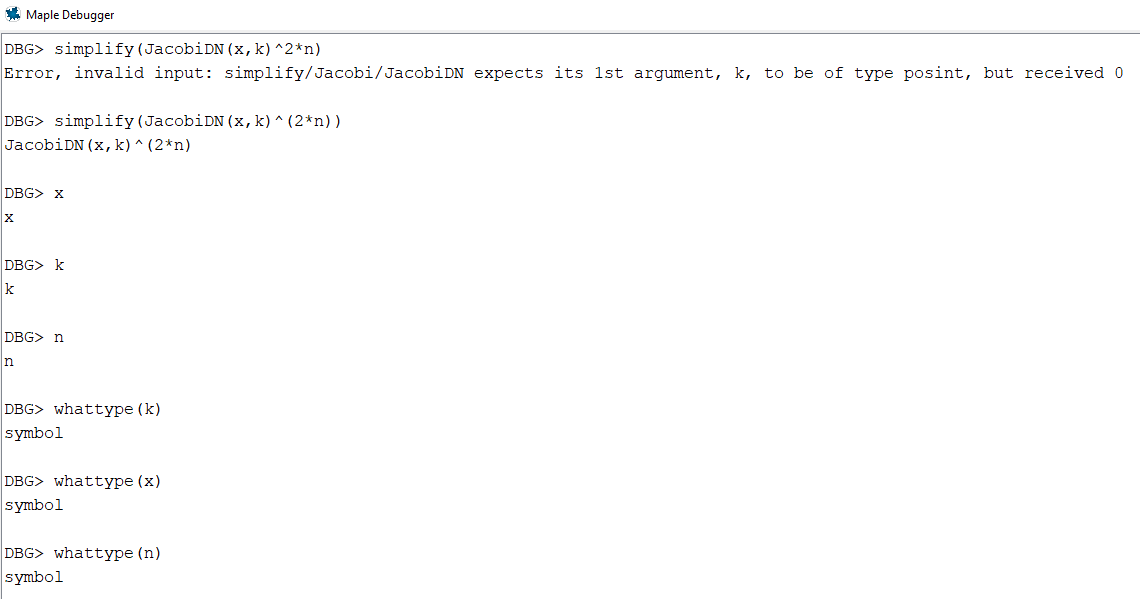# Question:invalid input: simplify/Jacobi/JacobiDN expects its 1st argument, k, to be of type posint, but received 0 error

## Question:invalid input: simplify/Jacobi/JacobiDN expects its 1st argument, k, to be of type posint, but received 0 error

Maple 2023

I am not able to make a MWE for this error, as it only shows in the debugger. So it seems Maple internal memory changes or some other library is loaded to cause this. Inside the debugger, I get to a function which does this

```DBG> simplify(JacobiDN(x,k)^2*n)

Error, invalid input: simplify/Jacobi/JacobiDN expects its 1st argument, k, to be of type posint, but received 0```

Version 2023 on windows 10

In a worksheet, the above works just fine

```restart;
simplify(JacobiDN(x,k)^2*n)```Back to the debugger, if I write (2*n) instead of 2*n, then the error goes away

```DBG> simplify(JacobiDN(x,k)^(2*n))
JacobiDN(x,k)^(2*n)```

The values of x,k,n are all symbols and have no values in the code running:I have no idea why this happend when I run the code only. It think x is zero in the above for some reason.

Sorry can't make MWE, I wish I can. Something strange happens when I run the code that does not show otherwise.

Any suggestions how to invetigate this more? Stepping into the simplify code it fails in

```DBG> next
`simplify/check_constant`:
3   return type(r,'And(constant,Or(Not(function),satisfies(f -> evalb(op(f)
<> NULL))))')

DBG> r
JacobiDN(x,k)^2*n^2

DBG> type(r,'And(constant,Or(Not(function),satisfies(f -> evalb(op(f)          <> NULL))))')
false

DBG> step
`simplify/Jacobi`
`simplify/do`:
84               userinfo(1,simplify,'applying',new_simp,
`function to expression`);

85               new_r := new_simp(r,symb_mode);

Here it generate the error.

```

It has nothing to do with simplify. Here is a call to integrate which gives same error

```DBG> lhs(ode)
diff(diff(xi(x),x),x)-k^2*JacobiSN(x,k)*JacobiCN(x,k)/JacobiDN(x,k)*diff(xi(x),x)+(-k^2*JacobiCN(x,k)^2+k^2*JacobiSN(x,k)^2-k^4*JacobiSN(x,k)^2*JacobiCN(x,k)^2/JacobiDN(x,k)^2-JacobiSN(x,k)^2*k^2*n^2+n^2)*xi(x)

DBG> int(lhs(ode),x)
Error, invalid input: simplify/Jacobi/JacobiDN expects its 1st argument, k, to be of type posint, but received 0

DBG> x
x

DBG> k
k

DBG> xi(x)
xi(x)
```﻿